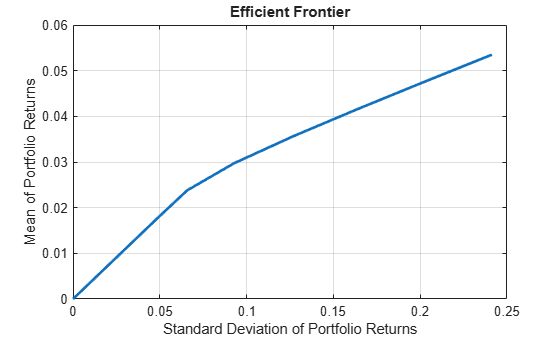# abs2active

Convert constraints from absolute to active format

## Syntax

``ActiveConSet = abs2active(AbsConSet,Index)``

## Description

example

````ActiveConSet = abs2active(AbsConSet,Index)` transforms a constraint matrix to an equivalent matrix expressed in active weight format (relative to the index).```

## Examples

collapse all

Set up constraints for a portfolio optimization for portfolio w0 with constraints in the form `A*w` <= `b`, where `w` is absolute portfolio weights. (Absolute weights do not depend on the tracking portfolio.) Use `abs2active` to convert constraints in terms of absolute weights into constraints in terms of active portfolio weights, defined relative to the tracking portfolio `w0`. Assume three assets with the following mean and covariance of asset returns:

```m = [ 0.14; 0.10; 0.05 ]; C = [ 0.29^2 0.4*0.29*0.17 0.1*0.29*0.08; 0.4*0.29*0.17 0.17^2 0.3*0.17*0.08;... 0.1*0.29*0.08 0.3*0.17*0.08 0.08^2 ];```

Absolute portfolio constraints are the typical ones (weights sum to `1` and fall from `0` through `1`), create the `A` and `b` matrices using `portcons`.

`AbsCons = portcons('PortValue',1,3,'AssetLims', [0; 0; 0], [1; 1; 1;]);`

Use the `Portfolio` object to determine the efficient frontier.

```p = Portfolio('AssetMean', m, 'AssetCovar', C); p = p.setInequality(AbsCons(:,1:end-1), AbsCons(:,end)); p.plotFrontier;```The tracking portfolio `w0` is:

`w0 = [ 0.1; 0.55; 0.35 ];`

Use `abs2active` to compute the constraints for active portfolio weights.

`ActCons = abs2active(AbsCons, w0)`
```ActCons = 8×4 1.0000 1.0000 1.0000 0 -1.0000 -1.0000 -1.0000 0 1.0000 0 0 0.9000 0 1.0000 0 0.4500 0 0 1.0000 0.6500 -1.0000 0 0 0.1000 0 -1.0000 0 0.5500 0 0 -1.0000 0.3500 ```

Use the `Portfolio` object `p` and its efficient frontier to demonstrate expected returns and risk relative to the tracking portfolio `w0`.

```p = p.setInequality(ActCons(:,1:end-1), ActCons(:,end)); p.plotFrontier;```Note, when using `abs2active` to compute “active constraints” for use with a `Portfolio` object, don't use the Portfolio object’s default constraints because the relative weights can be positive or negative (the `setDefaultConstraints` function for a `Portfolio` object specifies weights to be nonnegative).

## Input Arguments

collapse all

Portfolio linear inequality constraint matrix expressed in absolute weight format, specified as `[A b]` such that `A*w <= b`, where `A` is a number of constraints (`NCONSTRAINTS`) by number of assets (`NASSETS`) weight coefficient matrix, and `b` and `w` are column vectors of length `NASSETS`. The value `w` represents a vector of absolute asset weights whose elements sum to the total portfolio value. See the output `ConSet` from `portcons` for additional details about constraint matrices.

Data Types: `double`

Index portfolio weights, specified as a `NASSETS`-by-`1` vector. The sum of the index weights must equal the total portfolio value (for example, a standard portfolio optimization imposes a sum-to-1 budget constraint).

Data Types: `double`

## Output Arguments

collapse all

Transformed portfolio linear inequality constraint matrix expressed in active weight format, returned in the form `[A b]` such that `A*w <= b`. The value `w` represents a vector of active asset weights (relative to the index portfolio) whose elements sum to zero.

## Algorithms

`abs2active` transforms a constraint matrix to an equivalent matrix expressed in active weight format (relative to the index). The transformation equation is

`$A{w}_{absolute}=A\left({w}_{active}+{w}_{index}\right)\le {b}_{absolute}.$`

Therefore

`$A{w}_{active}\le {b}_{absolute}-A{w}_{index}={b}_{active}.$`

The initial constraint matrix consists of `NCONSTRAINTS` portfolio linear inequality constraints expressed in absolute weight format. The index portfolio vector contains `NASSETS` assets.